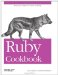# Converting Between Degrees and Radians

Problem

The trigonometry functions in Ruby's Math library take input in radians (2π radians in a circle). Most real-world applications measure angles in degrees (360 degrees in a circle). You want an easy way to do trigonometry with degrees.

Solution

The simplest solution is to define a conversion method in Numeric that will convert a number of degrees into radians.

```	class Numeric
def degrees
self * Math::PI / 180
end
end
```

You can then treat any numeric object as a number of degrees and convert it into the corresponding number of radians, by calling its degrees method. Trigonometry on the result will work as you'd expect:

```	90.degrees # => 1.5707963267949
Math::tan(45.degrees) # => 1.0
Math::cos(90.degrees) # => 6.12303176911189e-17
Math::sin(90.degrees) # => 1.0
Math::sin(89.9.degrees) # => 0.999998476913288

Math::sin(45.degrees) # => 0.707106781186547
Math::cos(45.degrees) # => 0.707106781186548
```

Discussion

I named the conversion method degrees by analogy to the methods like hours defined by Rails. This makes the code easy to read, but if you look at the actual numbers, it's not obvious why 45.degrees should equal the floating-point number 0.785398163397448.

If this troubles you, you could name the method something like degrees_to_radians. Or you could use Lucas Carlson's units gem, which lets you define customized unit conversions, and tracks which unit is being used for a particular number.

```	require 'rubygems'
require 'units/base'

class Numeric
remove_method(:degrees) # Remove the implementation given in the Solution
end

90.degrees # => 90.0
90.degrees.unit # => :degrees

```

The units you define with the units gem do nothing but make your code more readable. The trigonometry methods don't understand the units you've defined, so you'll still have to give them numbers in radians.

```	# Don't do this:
Math::sin(90.degrees) # => 0.893996663600558

# Do this:
```

Of course, you could also change the trigonometry methods to be aware of units:

```	class << Math
alias old_sin sin
def sin(x)
old_sin(x.unit == :degrees ? x.to_radians : x)
end
end

90.degrees # => 90.0
Math::sin(90.degrees) # => 1.0
Math::sin(Math::PI/2) # => 1.0
```

That's probably overkill, though.

• Recipe 8.9, "Converting and Coercing Objects to Different Types"
• The Facets More library (available as the facets_more gem) also has a Units moduleRuby Cookbook (Cookbooks (OReilly))
ISBN: 0596523696
EAN: 2147483647
Year: N/A
Pages: 399